# Peano axioms

(diff) ← Older revision | Latest revision (diff) | Newer revision → (diff)

A system of five axioms for the set of natural numbers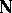and a function(successor) on it, introduced by G. Peano (1889):

1)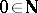;

2)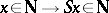;

3)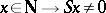;

4)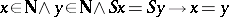;

5)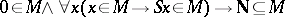for any property(axiom of induction).

In the first version 1 was used instead of 0. Similar axioms were proposed by R. Dedekind (1888). Peano's axioms are categorical, that is, any two systems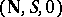and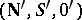satisfying them are isomorphic. The isomorphism is determined by a function, where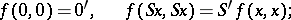The existence offor all pairs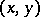and the mutual single-valuedness for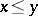are proved by induction. Peano's axioms make it possible to develop number theory; in particular, to introduce the usual arithmetic functions and to establish their properties. All the axioms are independent, but

and

can be combined to a single one:if one defines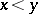as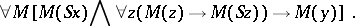The independence is proved by exhibiting a model on which all the axioms are true except one. For

such a model is the series of natural numbers beginning with 1; for

it is the set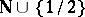, where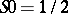,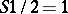; for

the set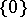; for

the setwith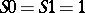; for

the set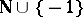.

Sometimes one understands by Peano arithmetic the system in the first-order language with the function symbols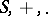, consisting of the axioms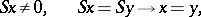defining equalities forand, and the induction scheme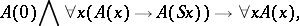whereis an arbitrary formula, known as the induction formula (see Arithmetic, formal).

How to Cite This Entry:
Peano axioms. Encyclopedia of Mathematics. URL: http://encyclopediaofmath.org/index.php?title=Peano_axioms&oldid=11715
This article was adapted from an original article by G.E. Mints (originator), which appeared in Encyclopedia of Mathematics - ISBN 1402006098. See original article